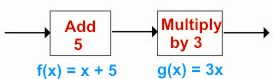HOME MATH DICTIONARY DOWNLOAD FEEDBACK DISCLAIMER
 Question: What is a Composite Function ? Answer: A composite function is a function which is composed of (made up from) a sequence of simple functions. For example, a composite function could be made up from the add 5 and the multiply by 3 functions. This can be represented by a machine chain.An input of 4 would give an output of f(4) = 4 + 5 = 9 from the first machine and then g(9) = 3 x 9 = 27 from the second machine In same way an input of x gives an output of f(x) = x + 5 from the first machine and then g(f(x)) = g(x + 5) = 3(x + 5) from the second machine. The composite function is x = 3(x + 5) and is symbolized by gf. When the two functions are combined the other way round, the composite function is given by f(g(x)) = f(3x) = 3x + 5. This function x = 3x + 5 is symbolized by fg. Note carefully the order: fg means first apply g, then apply f.